Data Structure

# Bellman-ford Algorithm

Bellman–Ford algorithm is an algorithm that solves the shortest path from a single source vertex to all of the other vertices in a weighted digraph.

Bellman-Ford algorithm returns a boolean value indicating whether or not there is a negative-weight cycle that is reachable from the source. If there is such a cycle, the algorithm indicates that no solution exists. If there is no such cycle, the algorithm produces the shortest paths and their weights.

This algorithm returns TRUE if and only if the graph contains no negativeweight cycles that are reachable from the source.

### Bellman-ford Algorithm

```BELLMAN-FORD(G, w, s)
1 INITIALIZE-SINGLE-SOURCE(G, s)
2 for i ← 1 to |V[G]| - 1
3 	do for each edge (u, v)  E[G]
4 		do RELAX(u, v, w)
5 for each edge (u, v)  E[G]
6 	do if d[v] > d[u] + w(u, v)
7 		then return FALSE
8 return TRUE```

### Example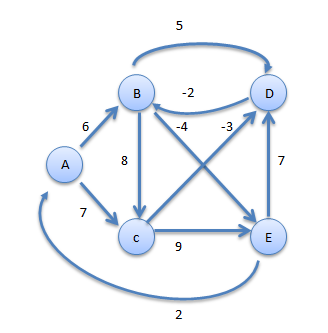Step1

#### Consider A as source vertex

 No. of Nodes A B C D E Distance 0 6 7 ∞ ∞ Distance From A A A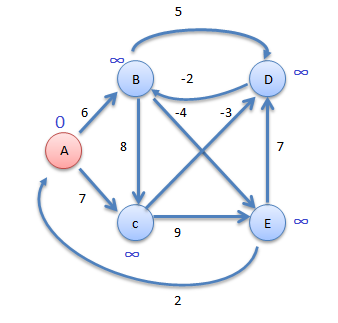Step2

#### Now consider vertex B

 No. of Nodes A B C D E Distance 0 6 7 11 2 Distance From A A A B BStep3

#### Now consider vertex E

 No. of Nodes A B C D E Distance 0 6 7 9 2 Distance From A A A E BStep4

#### Now consider vertex C

 No. of Nodes A B C D E Distance 0 6 7 4 2 Distance From A A A C B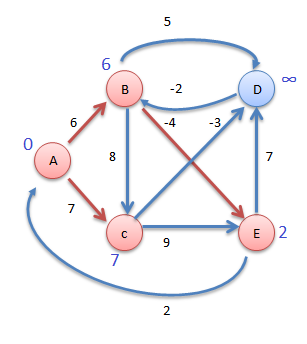Step5

#### Now consider vertex D

 No. of Nodes A B C D E Distance 0 2 7 4 2 Distance From A D A C B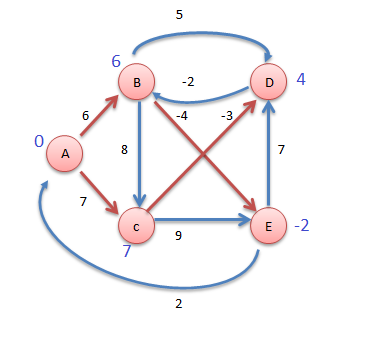Step6

#### Now consider vertex B

 No. of Nodes A B C D E Distance 0 2 7 4 -2 Distance From A D A C BStep7

#### Now consider vertex E

 No. of Nodes A B C D E Distance 0 2 7 4 -2 Distance From A D A C B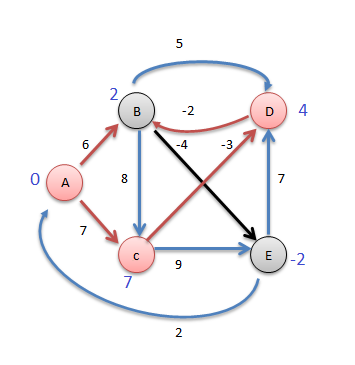Vertex Distance from A
A 0
B 2
C 7
D 4
E -2